Updating search results...

# 26 Results

View
Selected filters:
• NC.Math.8.F.3Conditional Remix & Share Permitted
CC BY-NC-SA
Rating
0.0 stars

This 8th grade Math&nbsp;parent guide&nbsp;explains the content in straightforward terms so they can support their children&rsquo;s learning at home and will&nbsp;encourage&nbsp;caretaker engagement&nbsp;with lessons.

Subject:
Mathematics
Material Type:
Reference Material
Author:
Kelly Rawlston
Letoria Lewis
01/10/2022Conditional Remix & Share Permitted
CC BY-NC-SA
Rating
0.0 stars

Our Teacher Guides are meant to support the use of our online course and unit content. Please use these to accompany the use of our content and for ideas to support struggling learners, those needing extension and for additional resources.

Subject:
Mathematics
Material Type:
Curriculum
Teaching/Learning Strategy
Author:
Kelly Rawlston
Letoria Lewis
05/16/2022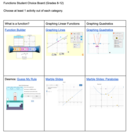Unrestricted Use
Public Domain
Rating
0.0 stars

Students will complete a Functions Choice Board they will have two options from each section. What is a function, graphing linear functions and Graphing Quadratic.

Subject:
Mathematics
Material Type:
Activity/Lab
Author:
Tyler Bridgers
02/24/2022Educational Use
Rating
0.0 stars

Unit on determining functions, comparing different representations of functions and graphing functions

Subject:
Mathematics
Material Type:
Activity/Lab
Provider:
Author:
04/23/2018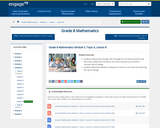Conditional Remix & Share Permitted
CC BY-NC-SA
Rating
0.0 stars

In this lesson, students examine the average rate of change for non-linear functions and learn that, unlike linear functions, non-linear functions do not have a constant rate of change. Students determine whether an equation is linear or non-linear by examining the rate of change.

Subject:
Mathematics
Material Type:
Lesson Plan
Provider:
EngageNY
02/21/2017Conditional Remix & Share Permitted
CC BY-NC
Rating
0.0 stars

In CK-12 Middle School Math Concepts Grade 8, the learning content is divided into concepts. Each concept is complete and whole providing focused learning on an indicated objective. Theme-based concepts provide students with experiences that integrate the content of each concept. Students are given opportunities to practice the skills of each concept through real-world situations, examples, guided practice and explore more practice. There are also video links provided to give students an audio/visual way of connecting with the content.

Subject:
Mathematics
Material Type:
Full Course
Lesson Plan
Textbook
Unit of Study
Provider:
CK-12 Foundation
Provider Set:
CK-12 FlexBook
12/06/2018Unrestricted Use
CC BY
Rating
0.0 stars

Function end of unit review function board.&nbsp;

Subject:
Mathematics
Material Type:
Activity/Lab
Author:
Tyler Bridgers
11/16/2021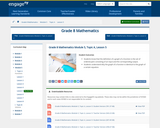Conditional Remix & Share Permitted
CC BY-NC-SA
Rating
0.0 stars

In this lesson, students know that the definition of a graph of a function is the set of ordered pairs consisting of an input and the corresponding output. Students understand why the graph of a function is identical to the graph of a certain equation.

Subject:
Mathematics
Material Type:
Lesson Plan
Provider:
EngageNY
02/21/2017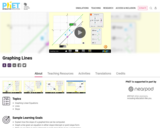Unrestricted Use
CC BY
Rating
0.0 stars

Sample Learning Goals
Explain how the slope of a graphed line can be computed.
Graph a line given an equation in either slope-intercept or point-slope form.
Write an equation in slope-intercept or point-slope form given a graphed line.
Predict how changing variables in a linear equation will affect the graphed line.

Subject:
Mathematics
Material Type:
Activity/Lab
Author:
PhET Interactive Simulations
10/05/2021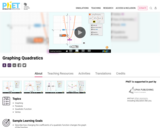Unrestricted Use
CC BY
Rating
0.0 stars

Sample Learning Goals
Describe how changing the coefficients of a quadratic function changes the graph of the function.
Predict how the graph of a parabola will change if the coefficients or constant are varied.
Identify the vertex, axis of symmetry, roots, and directrix for the graph of a quadratic equation.
Use the vertex form of a quadratic function to describe the graph of the function.
Describe the relationship between the focus and directrix and resulting parabola.
Predict the graph of a parabola given a focus and directrix.

Subject:
Mathematics
Material Type:
Activity/Lab
Author:
PhET Interactive Simulations
10/05/2021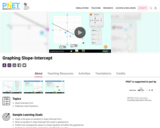Unrestricted Use
CC BY
Rating
0.0 stars

Sample Learning Goals
Graph a line given an equation in slope-intercept form.
Write an equation in slope-intercept form given a graphed line.
Predict how changing the values in a linear equation will affect the graphed line.
Predict how changing the graphed line will affect the equation.

Subject:
Mathematics
Material Type:
Activity/Lab
Author:
PhET Interactive Simulations
10/05/2021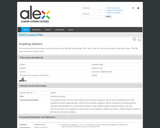Rating
0.0 stars

This activity will be used to review all the forms of linear equations and to review graphing linear, their parallels and their perpendiculars. All the forms of linear equations will be reviewed for testing purposes. Students will participate in a round robin activity to help students master objectives before unit test.

Subject:
Mathematics
Material Type:
Activity/Lab
Provider:
Alabama Learning Exchange
Author:
Sandra Kelley
02/26/2019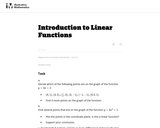Unrestricted Use
CC BY
Rating
0.0 stars

This task lets students explore the differences between linear and non-linear functions. By contrasting the two, it reinforces properties of linear functions. The task lends itself to an extended discussion comparing the differences that students have found and relating them back to the equation and the graph of the two functions.

Subject:
Mathematics
Material Type:
Activity/Lab
Provider:
Illustrative Mathematics
Provider Set:
Illustrative Mathematics
Author:
Illustrative Mathematics
08/08/2012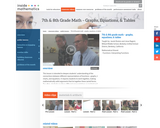Rating
0.0 stars

In this math video lesson on graphs and equations, students will get a deeper understanding of the connections between representations of functions, graphs, t-charts, and equations; they will make valid arguments that tie together these forms and show their application in solving problems. This resource supports English language development for English language learners.

Subject:
English as a Second Language
Mathematics
Material Type:
Lesson
Provider:
Inside Mathematics
Author:
Jacob Disston and Jesse Ragent
02/26/2019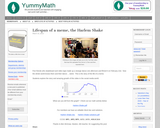Rating
0.0 stars

For this activity, students study data to answer questions.

Subject:
Mathematics
Material Type:
Activity/Lab
Provider:
YummyMath
04/27/2017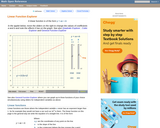Rating
0.0 stars

An interactive applet that allows the user to graphically explore the properties of a linear functions. Specifically, it is designed to foster an intuitive understanding of the effects of changing the two coefficients in the function y=ax+b. The applet shows a large graph of a quadratic (ax + b) and has two slider controls, one each for the coefficients a and b. As the sliders are moved, the graph is redrawn in real time illustrating the effects of these variations. Applet can be enlarged to full screen size for use with a classroom projector. This resource is a component of the Math Open Reference Interactive Geometry textbook project at http://www.mathopenref.com.

Subject:
Geometry
Mathematics
Material Type:
Simulation
Provider:
Math Open Reference
Author:
John Page
05/07/2019Rating
0.0 stars

The following materials include a Common Core-aligned task and formative assessments which may be integrated into a currently existing curriculum. The final task assesses student mastery of the standards related to linear functions.

Subject:
Mathematics
Material Type:
Activity/Lab
Assessment
Unit of Study
Provider:
The New York City Department of Education
Author:
The New York City Department of Education
02/26/2019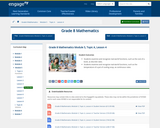Conditional Remix & Share Permitted
CC BY-NC-SA
Rating
0.0 stars

In this lesson, students examine and recognize real-world functions, such as the cost of a book, as discrete rates. Students examine and recognize real-world functions, such as the temperature of a pot of cooling soup, as continuous rates.

Subject:
Mathematics
Material Type:
Lesson Plan
Provider:
EngageNY
02/21/2017Rating
0.0 stars

For this task, students demonstrate how slopes are affected when reflections are made.

Subject:
Mathematics
Material Type:
Activity/Lab
Provider:
NRICH
03/23/2017Conditional Remix & Share Permitted
CC BY-NC-SA
Rating
0.0 stars

This course was created by the Rethink Education Content Development Team in partnership with the North Carolina Virtual Public Schools. This course is aligned to the NC Standards for 8th Grade Math.&nbsp;

Subject:
Mathematics
Material Type:
Activity/Lab
Assessment
Formative Assessment
Full Course
Homework/Assignment
Lecture Notes
Vocabulary
Author:
Kelly Rawlston
Letoria Lewis